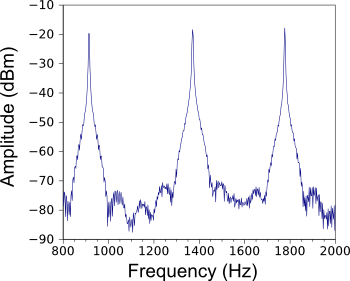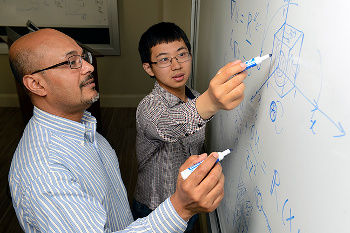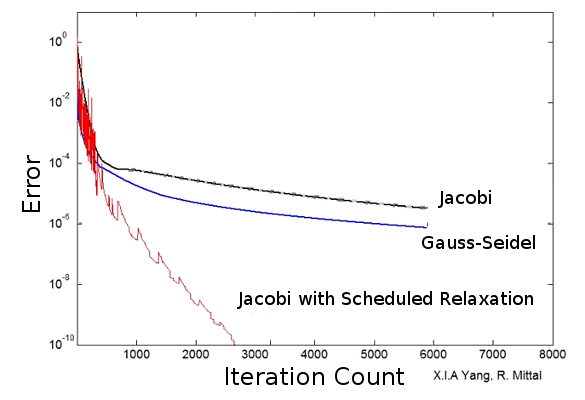### The Jacobi Method Updated

July 4, 2014

Mathematics is used extensively in science and engineering. Galileo, who was the first experimental physicist, also had the idea of using mathematics to express the results of his experiments. As he wrote in his 1623 book, The Assayer (Il Saggiatore),
Philosophy is written in this grand book, the universe... But the book cannot be understood unless one first learns to comprehend the language and read the letters in which it is composed. It is written in the language of mathematics, and its characters are triangles, circles, and other geometric figures without which it is humanly impossible to understand a single word of it; without these, one wanders about in a dark labyrinth."
Mathematicians have developed many things that are useful to scientists, but these things have become much more useful in our age of computers and technology. One example is the Fourier series. In 1811, Joseph Fourier showed that many periodic functions could be expressed as the sum of sine and cosine functions of integral fractions of the period.

Fourier originally developed his series in the study of thermal conduction. Today, the Fourier series has become especially useful as a means of signal analysis, as a way to separate signals into components for effective filtering, and as a method of data compression.

The broader implementation of the Fourier series was facilitated by the development of a rapid algorithm for its calculation. The fast Fourier transform (FFT) algorithm was invented in 1965 by James Cooley and John Tukey. the Cooley-Tukey FFT is a recursive algorithm that breaks the Fourier transform into smaller transforms that are calculated separately and combined to obtain the transform. As I wrote in a previous article (Moona Lisa, January 28, 2013), it was found that the essential trick of the FFT algorithm was discovered by Gauss around 1805 and published after his death.Frequency spectrum of one of the telephone system special information tones.

This FFT spectrum was obtained using the free and open source (FOSS) application, Audacity.

(Plot of Audacity data using Gnumeric.)

One thing done frequently by engineers is solving systems of linear equations. An early technique developed for doing this was the Jacobi method, invented in 1845 by Carl Gustav Jacob Jacobi (1804-1851), a German mathematician who was notably the first Jewish mathematics professor at a German university. The interesting thing about this method is that it starts with a guess of the solutions. It then iterates to the proper solution values. As in all iterative methods, which are programmed on computers using loops, the Jacobi method takes a while to find a solution.

The Jacobi method is rarely used today, except for small linear equation systems where the computer run-time is still short. It was replaced in the days before computers by other methods, such as the Gauss–Seidel method, which is almost an order of magnitude faster. As can be seen in just this short article, in which I mentioned his role in the fast Fourier transform, Gauss had his hand in much early mathematics.

At the Johns Hopkins University, Rajat Mittal, a professor in the Department of Mechanical Engineering, was summarizing the Jacobi method in a graduate class in numerical methods in 2012. His conclusion, which is the consensus of those in the field, was that other numerical methods are faster and better. One student in the class, Xiang Yang, at that time a first-year graduate student, became interested in the method. Yang experimented with making the Jacobi method more efficient. Eventually, Yang and Mittal arrived at an algorithm that gave results 200 times faster.[3-5]Graduate student, Xiang Yang, and Professor Rajat Mittal at a whiteboard.

An idea by Yang led to an improvement of the 169-year-old Jacobi method.

(Johns Hopkins University photo by Will Kirk.)[5)]

A paper on their method, which they call the "scheduled relaxation Jacobi method," will appear in the Journal of Computational Physics.[3-4] Says Rajat Mittal, "Our paper provides the recipe for how to speed up this method significantly by just changing four or five lines in the computer code." Mittal expects that this method will be quickly implemented for fluid mechanics calculations, such as those for airframe design. The method is apparently well suited for computation on parallel computers.Comparison of linear equation iterative methods of solution. These data are for the solution of the two-dimensional Laplace equation on a 128x128 grid. (Still image from a YouTube Video, modified for clarity.)[6)]

Yang, who received his undergraduate engineering degree at Peking University, is doing his doctoral research on a different topic, entirely. This is the affect of barnacles on ship hydrodynamics. The Jacobi method research was supported by the Office of Naval Research and the National Science Foundation.

### References:

Linked Keywords: Mathematics; science; engineering; Galileo Galilei; experiment; experimental; physicist; The Assayer; natural philosophy; book; universe; language; alphabet letter; triangle; circle; geometry; geometric; labyrinth; mathematician; scientist; computer; technology; Fourier series; Joseph Fourier; periodic function; sine; cosine; integer; fraction; thermal conduction; signal processing; signal analysis; electronic signal; filter; data compression; algorithm; fast Fourier transform; James Cooley; John Tukey; recursive algorithm; Fourier transform; Carl Friedrich Gauss; special information tone; free and open source; FOSS; Audacity; Gnumeric; engineer; linear equation; Jacobi method; Carl Gustav Jacob Jacobi (1804-1851); German; Jewish; professor; university; iteration; iterate; computer programming; control flow; loop; computer run-time; Gauss–Seidel method; Johns Hopkins University; Rajat Mittal; professor; Department of Mechanical Engineering; graduate school; numerical analysis; numerical method; Xiang Yang; postgraduate education; graduate student; efficiency; efficient; whiteboard; Will Kirk; scientific paper; Journal of Computational Physics; fluid mechanics; calculation; airframe; design; parallel computing; parallel computer; two-dimensional space; two-dimensional; Laplace equation; grid; YouTube Video; undergraduate engineering degree; Peking University; Doctor of Philosophy; doctoral; research; barnacle; ship; hydrodynamics; Office of Naval Research; National Science Foundation; J.W. Cooley and J.W. Tukey, "An algorithm for the machine calculation of complex Fourier," Math. Comput., vol. 19 (1965), pp. 297-301.